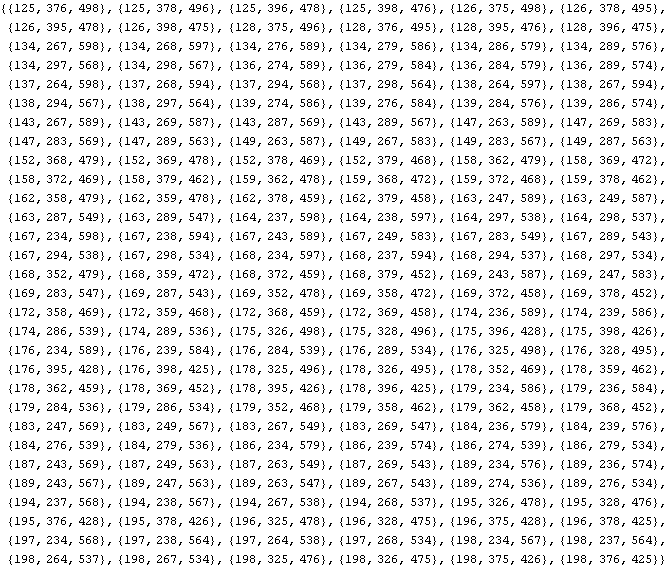# Three Columns, Using Numbers 1-9 To Equal 999. Can You Help?

It is really easy you need to make the first number 19 the second one 18 and the third one 9

thanked the writer.
The digits 1-9 total to 45. The digits in the sum you seek (999) total 27, which is 18 less than 45. This means there must be two columns in the sum that have a carry in. So, the left of the three columns must have digits that total to 8, the middle column must have digits that total to 18, and the right column of the three must have digits that total to 19. (8+18+19 = 45).

Three digits that total to 19 are 9+8+2. Three different digits that total to 18 are 7+6+5. The remaining (unused) digits are 4+3+1, which total to 8.

Thus, one possible set of numbers is
479
368
152
999

There are 5 different ways to sum 3 of the digits 1-9 so they total 19:
(9,8,2), (9,7,3), (9,6,4), (8,7,4), (8,6,5). For each of these, there is exactly one way to choose digits that total 18. In order, they are
(7,6,5), (8,6,4), (8,7,3), (9,6,3), (9,7,2). Of course, each of these sets has a corresponding set that totals 8:
(4,3,1), (5,2,1), (5,2,1), (5,2,1), (4,3,1).

Each of the 3 digits in a column can be permuted into 6 different sequences. So, there are 63*5 = 1080 different ways to write the sum to 999. If you only want to count different combinations of 3-digit numbers, then that total is divided by 6: There are 180 different sets of 3-digit numbers comprised of the digits 1-9 that total 999.

The picture lists all 180 sets.thanked the writer.